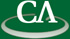## Parallel Sparse Polynomial Interpolation over Finite Fields

#### Mahdi Javadi, School of Computing Science, SFU.

Wednesday July 7th, in K9509 at 3:30pm.

```Abstract.

We present a probabilistic algorithm to interpolate a sparse multivariate
polynomial over a finite field, represented with a black box.  Our algorithm
modifies the algorithm of Ben-Or and Tiwari from 1988 for interpolating
polynomials over rings with characteristic zero to characteristic \$p\$ by

To  interpolate a polynomial in n variables with t non-zero terms, Zippel's
algorithm interpolates one variable at a time using O(ndt) probes to the
black box where d bounds the degree of the polynomial.  Our new algorithm
does O(nt) probes.  It interpolates each variable independently using O(t)
probes which allows us to parallelize the main loop giving an advantage
over Zippel's algorithm.

We have implemented both Zippel's algorithm and the new algorithm in C
and we have done a parallel implementation of our algorithm using Cilk.
In the talk we provide benchmarks comparing the number of probes our
algorithm does with both Zippel's algorithm and Kaltofen and Lee's hybrid
of the Zippel and Ben-Or/Tiwari algorithms.  The benchmarks also
demonstrate how effective our parallel implementation is.
```